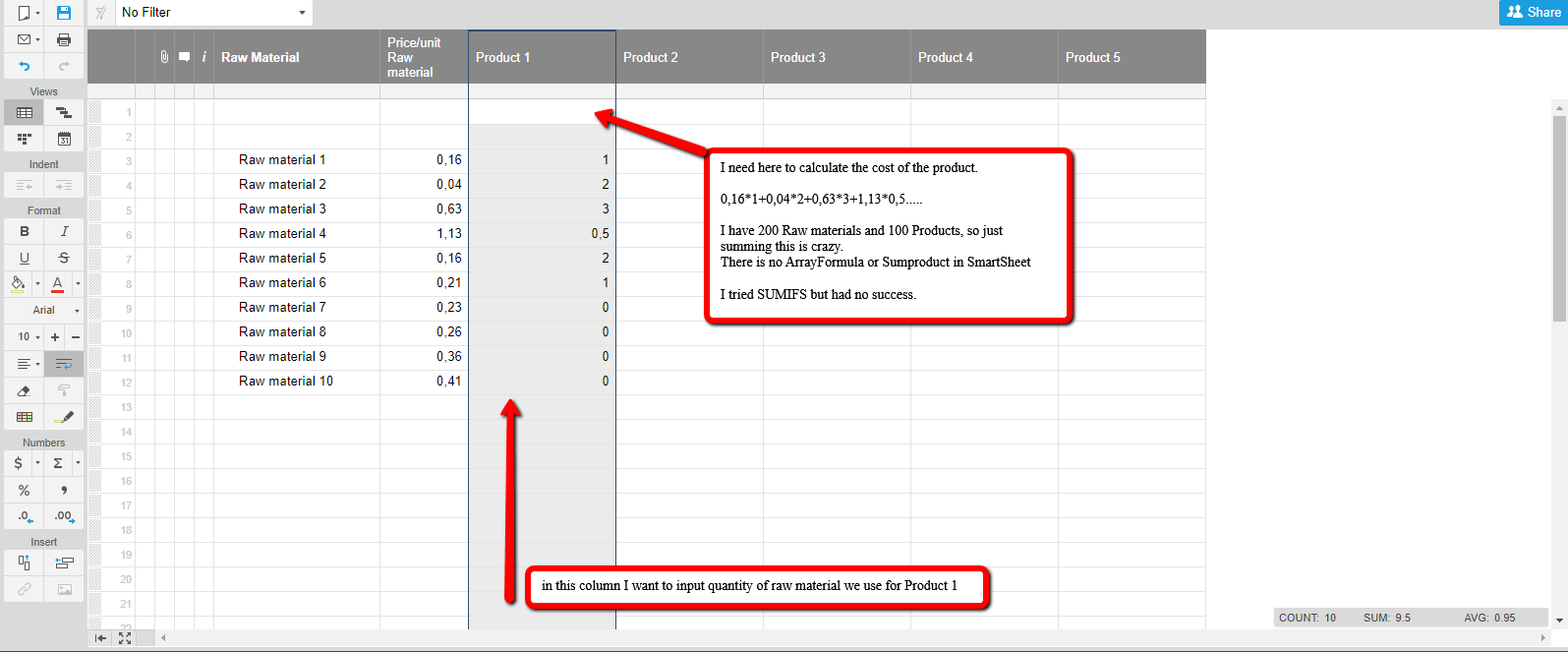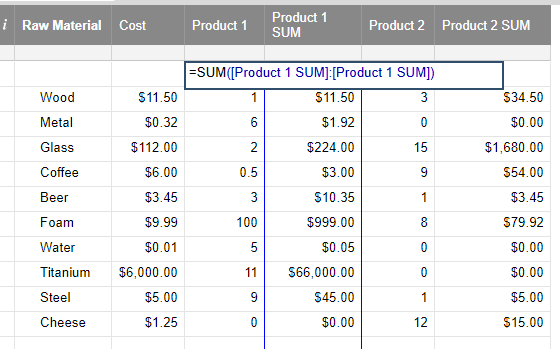# If no Array formula and no Sumproduct, what can I use?

I have been trying to create a product cost calculator where every column is a finished product and every row is a raw material. I am really bad with formulas but I had success with both Arrayformula and Sumproduct in Google Spreadsheets. But I need my data in Smartsheet and I can not calculate the cost of one product. Do you have any ideea on what should I use? Maby an example? ThanksTags:

• Hi Sorin,

You don't have access to arrays or sumproducts in Smartsheet, but there are workarounds.

Looking at your sheet, the big issue you will have is the placement of your total product cost in the first row of each [Product #] column. Smartsheet only adds new rows (beyond the initial 50 when a sheet is created) when you populate all the existing rows. As an example, you cannot create a formula that looks at [Product 1]\$2 through to [Product 1]\$5000 without first creating those additional 4950 rows (which is a pain).

It won't be so bad if you are only creating 200 rows but bear in mind that you'd need to update all your formulas (e.g. [Product 1]\$2:[Product 1]\$250) if you add extra rows beyond the 200.

Normally you would get around this by using a [Product 1]:[Product 1] range (as your image suggests). But here is where it's getting tricky, as your SUM at the top is part of the range and it will throw a circular reference error as it is including itself in the calculation (confusing huh?)

You'll need to think about how you want to structure your sheet(s) to address this. Enough of the waffling though. As you don't have the same formulas you did in Google Sheets, I'd suggest creating an additional column (that can be hidden) summing each product row (see image below).

The [Product 1]1 cell with ~\$67K has the following formula:

=SUM([Product 1 SUM]:[Product 1 SUM])

From row 2 down, the [Product 1 SUM] column has the following formula:

=\$Cost2 * [Product 1]2

The \$ sign represents an absolute value so when the formula is copied elsewhere (i.e. other columns) it still keeps looking at the Cost column.## Help Article Resources

Want to practice working with formulas directly in Smartsheet?

Check out the Formula Handbook template!